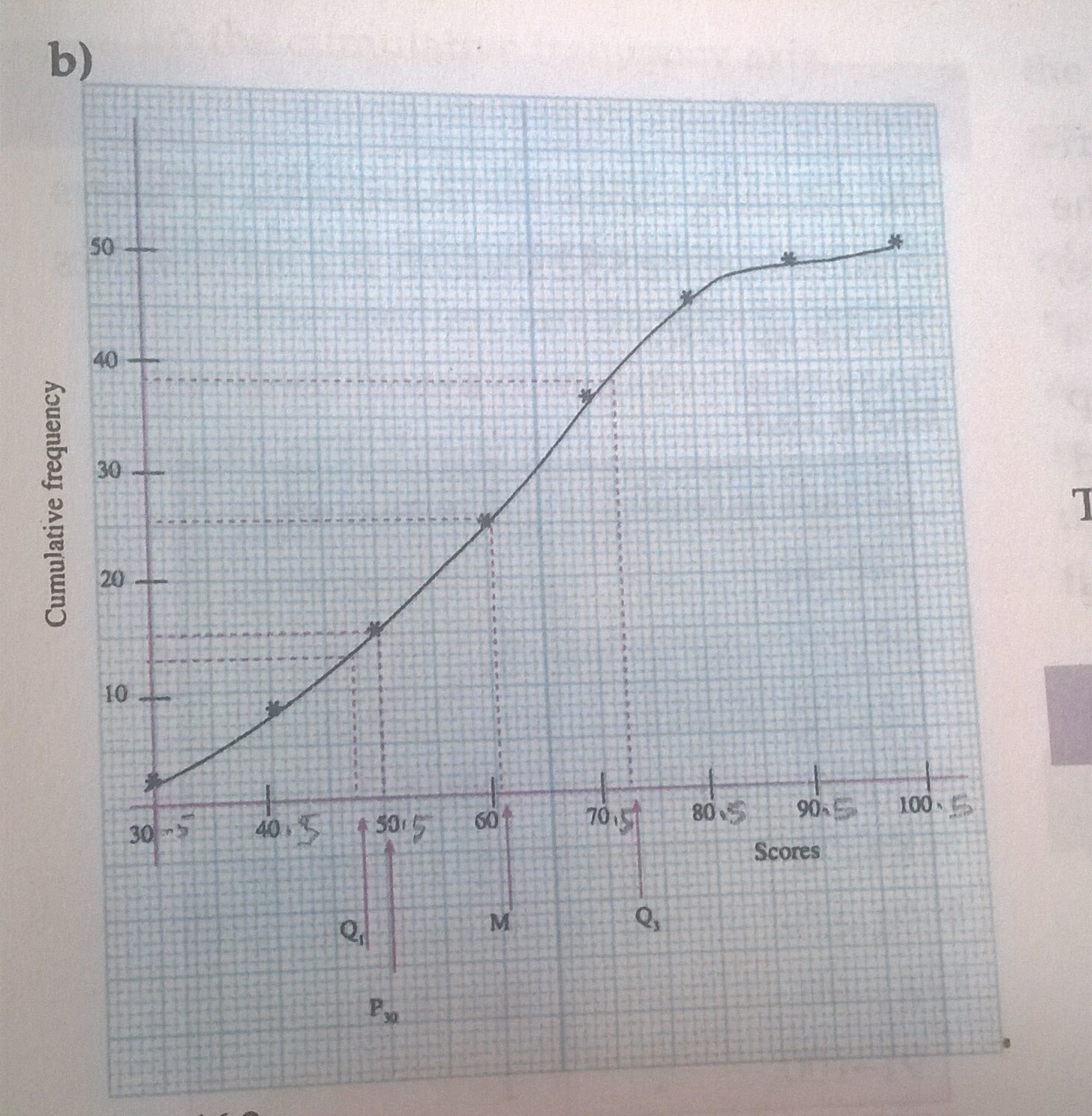Lesson 3&4

Class:SS2 & SS3

Subject: Mathematics

Topic: Cumulative Frequency Graph (Ogive)

It has been stated earlier in our previous lesson that the sum of all frequencies from the first class to a particular class is referred to as the cumulative frequency of that class. The graph of cumulative frequency distribution is a cumulative frequency curve which is also called the ogive.

The cumulative frequency graph has an elongated S – shape curve. It is obtained by plotting cumulative frequency on the y-axis against the corresponding upper class boundary on x-axis.

The cumulative frequency curve ( ogive) can be used to find the median, quartile, percentiles, deciles and interquartile range etc.

Determination of median, quartiLEs, deciles and percentiles from cumulative frequency graph.

1. 1. Median: This is the score that corresponds to the middle score half way up the cumulative frequency axis. It is also called the second quartile Q2. It is given by 1/2*N score on the cumulative frequency axis, where N is the total frequency.

The median can be determined as follows:

1. Determine half of the total frequency. That is 1/2*N
2. Locate this point on the cumulative frequency axis on the graph.
3. Through this located point on the cumulative frequency axis, draw a line parallel to the x-axis to meet the curve.
4. From this point of intersection, draw a perpendicular line to the x- axis.
5. Locate and determine the value of the point at which this vertical line meets the x- axis. This gives the median.

2.Quartile: The quartile is an average that divides the whole distribution (data) into four equal parts namely:

First or lower quartile (Q1): Is one- quarter up the cumulative frequency axis of the graph. Assuming the total frequency of a given distribution is N, then the lower quartile Q1 is given by 1/4*N score.

The lower quartile is determine from cumulative frequency graph as follows:

1. Determine one-quarter of the total frequency. That is 1/4*N

Repeat steps b to e as in (1) above to obtain the lower quartile.

upper quartile (Q3) is the third quartile of the distribution. As the name implies, it is three- quarter up the cumulative frequency axis of the graph. The upper quartile Q3 is given by 3/4*N score on the graph. The upper quartile is obtained from cumulative frequency graph as follows:

1. Determine three-quarter of the total frequency. That is 3/4*N

Repeat steps b to e as in (1) above to obtain the upper quartile Q3.

Interquartile range is the difference between the upper quartile and lower quartile values written as Q= Q3 – Q1.

semi-Interquartile range is the Interquartile range divided by two. That is

(Q3 -Q1)/2.

1. 3. Decile: Decile divides the data into ten equal parts. For instance, the 7th decile is 7/10*N. It is obtained from cumulative frequency graph as follows:
2. Determine 7/10*N on cumulative frequency axis

Repeat steps b to e as in (1) above to obtain the 7th decile.

1. 4. Percentile: Just like the quartile and decile, the percentile divides the data into hundred equal parts. For instance, the 30th percentile is 30/100*N. It is obtained from cumulative frequency graph as follows:
2. Determine 30/100*N on cumulative frequency axis

Repeat steps b to e as in (1) above to obtain the 30th percentile

Example 1: The following shows the distribution of scores obtained by SS2 students in mathematics mock exam.

 Class interval 21-30 31-40 41-50 51-60 61-70 71-80 81-90 90-100 Frequency 2 6 7 10 11 9 4 1
1. Construct a cumulative frequency table for the distribution.
2. Draw a cumulative frequency curve for the distribution.
3. From your graph, estimate the:
4. median
5. interquartile range

iii. pass mark, if 70% of the students passed.

.                       Solution

a.

 Class interval Frequency Class boundary Cumulative frequency 21-30 2 20.5-30.5 2 31-40 6 30.5-40.5 8 41-50 7 40.5-50.5 15 51-60 10 50.5-60.5 25 61-70 11 60.5-70.5 36 71-80 9 70.5-80.5 45 81-90 4 80.5-90.5 49 91-100 1 90.5-100.5 501. ci.

Given N=50,

Recall that, median= 1/2*N. That is 1/2*50=25

From the graph, the median =63  (That is 25 on the Y-axis corresponds to 63 on the x-axis on the graph)

cii.

Q3 =3/4*N, That is 3/4*50=37.5

From the graph, Q3=73

Q1 =1/4*N, That is 1/4*50=12.5

From the graph, Q1=48.

Hence the interquartile range(Q) =Q3-Q1

Q=75-48

=25

ciii.

If 70% of the students passed the exam, it means 30% failed. We therefore, need to find only 30th percentile on the cumulative frequency graph; above this point we have the 70% needed. That is

30th percentile (P30) =30/100*N

P30=30/100*50=15

From the graph, P30=50.5

Therefore the pass mark is 50.5

Assignment

The table below shows the frequency distribution of marks scored by 120 students in Mathematics test.

 Marks% 10-19 20-29 30-39 40-49 50-59 60-69 70-79 80-89 Frequency 23 19 6 25 15 11 12 9
1. Prepare a cumulative frequency table and use it to draw the ogive for the data.
2. Use your graph to estimate the:
3. lower quartile
4. 70th percentile
5. Find the pass mark if 35 candidates passed the exam. (Hint: Get the pass mark from the y-axis)